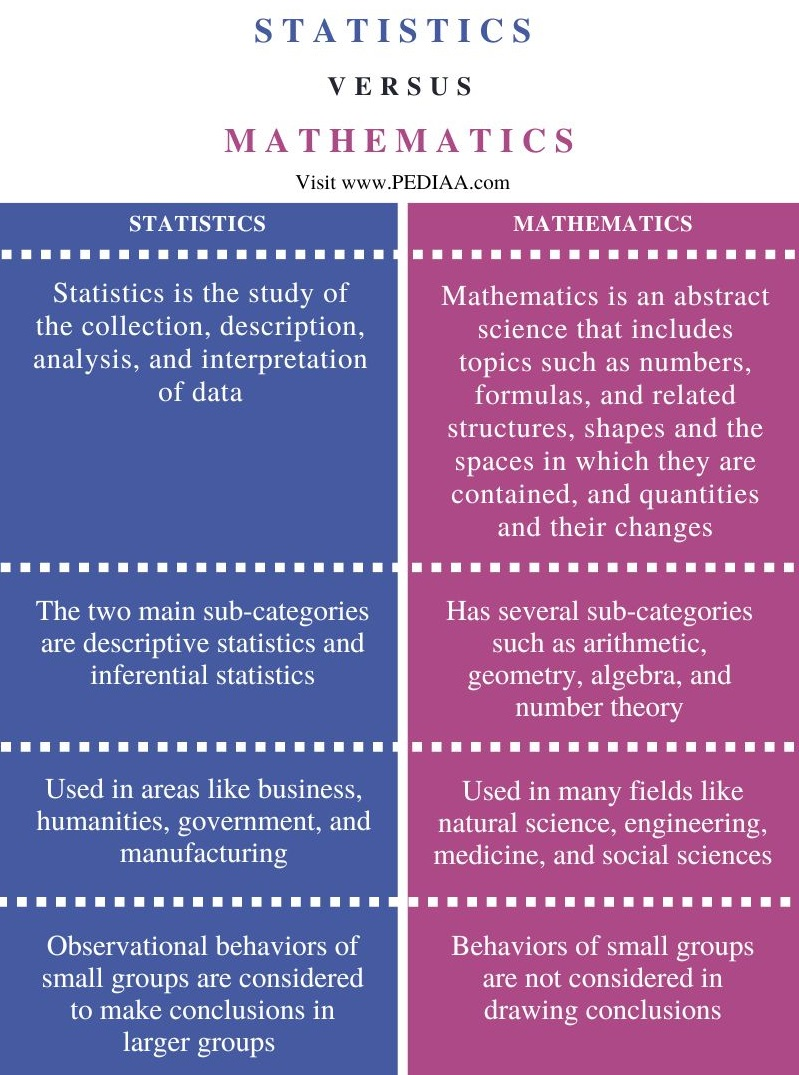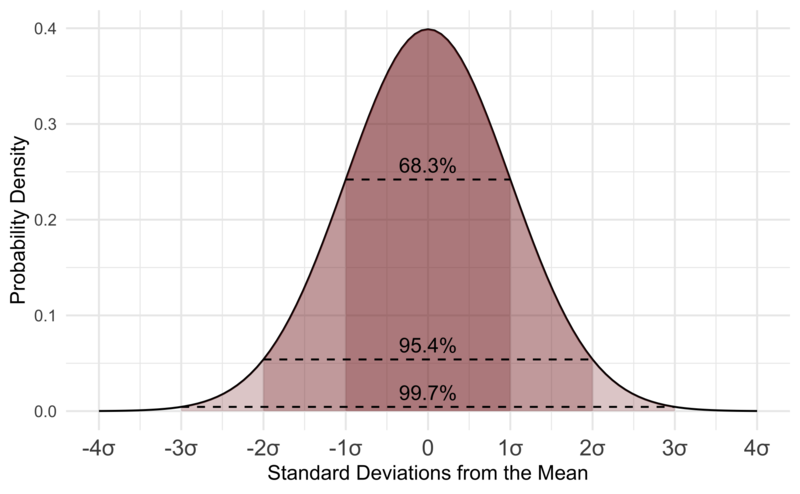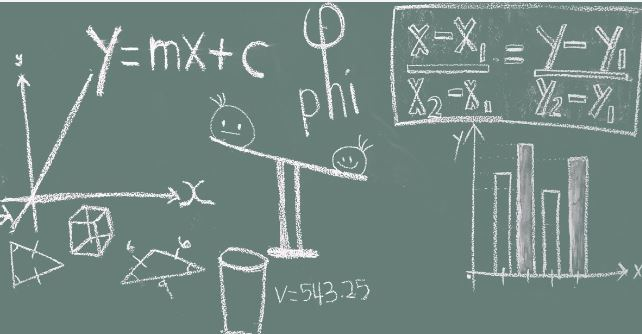# What is the Difference Between Statistics and Mathematics

The main difference between mathematics and statistics is that statistics is a study of collection, description, analysis, interpreting, and representation of data, while mathematics is the abstract science of number, quantity, and space.

Although both statistics and mathematics deal with numbers and digits, there are considerable differences between the two fields.

### Key Areas Covered

1. What is Statistics
– Definition, Features
2. What is Mathematics
– Definition, Features
2. Similarities Between Statistics and Mathematics
– Outline of Common Features
3. Difference Between Statistics and Mathematics
– Comparison of Key Differences

### Key Terms

Mathematics, Statistics## What is Statistics

Statistics is a branch of applied mathematics and is a study of the collection, description, analysis, and interpretation of data. Statistics is heavily used in scientific fields and research contexts. In statistics, the conclusions are made in larger groups by simply considering the characteristics and observable behaviors of smaller groups. Thus, these samples represent a portion of the larger groups.There are two major areas in statistics. They are descriptive statistics and inferential statistics. Descriptive statistics describe the properties of sample and population data, while inferential statistics uses these properties to test hypotheses and draw conclusions. Descriptive statistics describe or summarize the characteristics of a sample or data set, such as a variable’s mean, standard deviation, or frequency. On the other hand, inferential statistics are used to employ any number of techniques to relate variables in a data set to one another; for instance, using correlation or regression analysis. Then these can be used to estimate forecasts or infer causality. At the same time, there are statistical tools such as descriptive, mean, variance, inferential, and linear regression analysis. Furthermore, statistics is useful in social sciences, business, humanities, government, and manufacturing. There are two fundamental ideas in the field of statistics as uncertainty and variation.

## What is Mathematics

Mathematics is the science of number, quantity, and space, evolved from the basic practices of counting, measuring, and systematic study of shapes and motions. Mathematicians look for patterns, formulate new conjectures, and establish the truth. We can describe mathematics as a combination of logical reasoning and quantitative calculation. Mathematics is an essential tool in many vital fields like natural science, engineering, medicine, and the social sciences. Moreover, applied mathematics is a branch of mathematics that concerns the application of mathematical knowledge in other fields and new mathematical discoveries. Most mathematical activities involve discovering and proving the properties of abstract objects by pure reasoning.There are many branches of mathematics, such as arithmetic, geometry, number theory, algebra, and discrete mathematics. Number theory deals with natural numbers, whereas geometry deals with shapes such as lines, angles, and circles. Algebra, on the other hand, focuses on the formulations of equations and formulas. Overall, all these branches of mathematics can help in our daily lives.

## Similarities Between Statistics and Mathematics

• Both statistics and mathematics are helpful in many different fields.
• Statistics and mathematics use numbers and digits.

## Difference Between Statistics and Mathematics

### Definition

Statistics is a study of the collection, description, analysis, and interpretation of data, while mathematics is an abstract science that includes topics such as numbers, formulas, and related structures, shapes and the spaces in which they are contained, and quantities and their changes.

### Sub-categories

The two main sub-categories of statistics are descriptive statistics and inferential statistics, while mathematics has several sub-categories such as arithmetic, geometry, algebra, and number theory.

### Uses

Although statistics is used in areas like business, humanities, government, and manufacturing, mathematics is used in many fields like natural science, engineering, medicine, and the social sciences.

### Representation

In statistics, observational behaviors of small groups are considered to make conclusions in larger groups whereas in mathematics, the behaviors of small groups are not considered in drawing conclusions.

### Conclusion

Mathematics is an abstract science that focuses on the understanding of space, measure, and study of quality, whereas statistics is a branch of applied science that focuses on the collection, sorting, and representation of data. Thus, this is the main difference between mathematics and statistics.

##### Reference:

1. “Mathematics.” Encyclopædia Britannica, Encyclopædia Britannica, Inc.
2. “Statistics.” Wikipedia, Wikimedia Foundation.
3. “What Is Mathematics.” Tennessee Tech University.

##### Image Courtesy:

1. “Standard Normal Distribution” By D Wells – Own work (CC BY-SA 4.0) via Commons Wikimedia
2. “Photo of number, line, blackboard, scale, math, education, classroom, equation, chalkboard, brand, font, study, sketch, drawing, chalk, illustration, text, diagram, mathematics, school, learn, learning, lesson, formula, teach” (CC0) via Pxhere